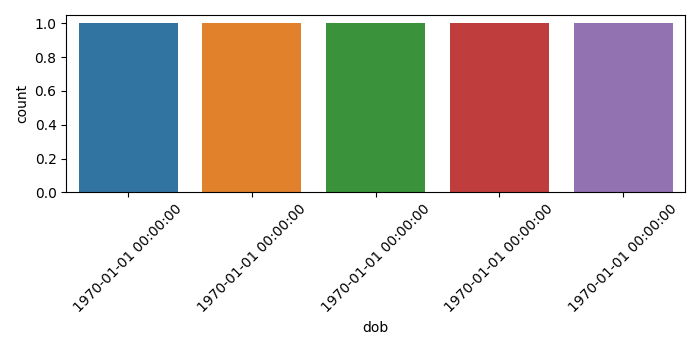# How to plot int to datetime on X-axis using Seaborn?

To plot int to datetime on X-axis using Seaborn in matplotlib, we can take the following steps

## Steps

• Set the figure size and adjust the padding between and around the subplots.

• Create a dataframe, df, of two-dimensional, size-mutable, potentially heterogeneous tabular data, with three columns.

• Create a countplot with int, i.e., dob on the X-axis.

• Set int to datetime label on the X-axis.

• To display the figure, use Show() method.

## Example

import seaborn as sns
from matplotlib import pyplot as plt
import pandas as pd
import numpy as np

# Set the figure size
plt.rcParams["figure.figsize"] = [7.00, 3.50]
plt.rcParams["figure.autolayout"] = True

# Data frame with 3 columns
df = pd.DataFrame([
[23, 'James', 1259969793926],
[39, 'Jimmy', 1259969793927],
[56, 'Jack', 1259969793929],
[60, 'Tom', 1259969793928],
[80, 'Tim', 1259969793939]
], columns=['marks', 'names', 'dob'])

# Create a Countplot with df
ax = sns.countplot(x="dob", data=df)

ax.set_xticklabels(
[
pd.to_datetime(tm, unit='ms').strftime('%Y-%m-%d %H:%M:%S')
for tm in ax.get_xticks()
], rotation=45)

# Display the plot
plt.show()

## Output

It will produce the following output −